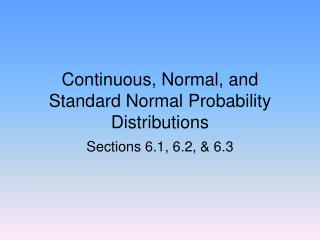DownloadDownload PresentationContinuous, Normal, and Standard Normal Probability Distributions

# Continuous, Normal, and Standard Normal Probability Distributions

Download Presentation## Continuous, Normal, and Standard Normal Probability Distributions

- - - - - - - - - - - - - - - - - - - - - - - - - - - E N D - - - - - - - - - - - - - - - - - - - - - - - - - - -
##### Presentation Transcript

1. Continuous, Normal, and Standard Normal Probability Distributions Sections 6.1, 6.2, & 6.3

2. Continuous Random Variables • Not countable. • Examples: • Weights of babies. • Heights of women. • Amount paid for a house.

3. Probability Histograms and Curves • 0 ≤ P(X) ≤ 1 • Sum of all probabilities of intervals = 1

4. Probability of Intervals • P(X=65) =0

5. The Normal Distribution • Many natural random phenomenon have normal distributions. • Bell-shaped. • Area under curve = 1. • Curve is symmetric about the mean. • Tails extend indefinitely.

6. Normal Probability Curve • Parameters are µ and σ.

7. Mean and Standard Deviation

8. The Standard Normal Distribution • The mean = 0 and the standard deviation = 1. • Z-values or z-scores represent the values on the x-axis.

9. Find probabilities under the standard normal distribution • Find the area (probability) to the left of z = 1.95

10. Table IV in Appendix C

11. Area (Probability)

12. Example: • Find the area (Probability) z = -2.17 to z = 0

13. Example: • Find the area to the right of 2.32

14. Example: • Find the area between -1.56 and 2.31. • Check the Empirical Rule.